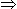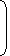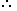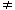# Tricks to Solve Logarithm Questions1. Logarithm:If a is a positive real number, other than 1 and am = x, then we write:
m = logax and we say that the value of log x to the base a is m.Examples:(i). 103 1000log10 1000 = 3.(ii). 34 = 81log3 81 = 4.

 (iii). 2-3 = 1log2 1 = -3. 8 8

(iv). (.1)2 = .01log(.1) .01 = 2.

2. Properties of Logarithms:1. loga (xy) = loga x + loga y
 2. logax= loga x – loga y y

3. logx x = 1

4. loga 1 = 0

5. loga (xn) = n(loga x)

 6. loga x = 1 logx a
 7. loga x = logb x = log x . logb a log a
3. Common Logarithms:Logarithms to the base 10 are known as common logarithms.
4. The logarithm of a number contains two parts, namely ‘characteristic’ and ‘mantissa’.Characteristic: The internal part of the logarithm of a number is called its characteristic.Case I: When the number is greater than 1.In this case, the characteristic is one less than the number of digits in the left of the decimal point in the given number.Case II: When the number is less than 1.

In this case, the characteristic is one more than the number of zeros between the decimal point and the first significant digit of the number and it is negative.

Instead of -1, -2 etc. we write 1 (one bar), 2 (two bar), etc.

Examples:-

 Number Characteristic Number Characteristic 654.24 2 0.6453 1 26.649 1 0.06134 2 8.3547 0 0.00123 3

Mantissa:

The decimal part of the logarithm of a number is known is its mantissa. For mantissa, we look through log table

1.Which of the following statements is not correct?
 A. log10 10 = 1 B. log (2 + 3) = log (2 x 3) C. log10 1 = 0 D. log (1 + 2 + 3) = log 1 + log 2 + log 3

Explanation:

(a) Since loga a = 1, so log10 10 = 1.

(b) log (2 + 3) = log 5 and log (2 x 3) = log 6 = log 2 + log 3log (2 + 3)log (2 x 3)

(c) Since loga 1 = 0, so log10 1 = 0.

(d) log (1 + 2 + 3) = log 6 = log (1 x 2 x 3) = log 1 + log 2 + log 3.

So, (b) is incorrect.

2. If log 2 = 0.3010 and log 3 = 0.4771, the value of log5 512 is:
 A. 2.87 B. 2.967 C. 3.876 D. 3.912

Explanation:

log5 512
 = log 512 log 5
 = log 29 log (10/2)
 = 9 log 2 log 10 – log 2
 = (9 x 0.3010) 1 – 0.3010
 = 2.709 0.699
 = 2709 699
= 3.876

#### If log 27 = 1.431, then the value of log 9 is:

Correct! Wrong!

#### If loga/b +logb/a = log (a + b), then:

Correct! Wrong!

#### If log10 2 = 0.3010, then log2 10 is equal to

Correct! Wrong!

#### If log10 2 = 0.3010, the value of log10 80 is:

Correct! Wrong!

#### If log10 5 + log10 (5x + 1) = log10 (x + 5) + 1, then x is equal to

Correct! Wrong!

logarithms quiz
GREAT JOB